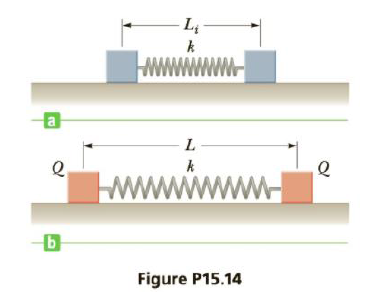Chapter 15, Problem 14P

Chapter
Section
Textbook Problem

Two identical metal blocks resting on a frictionless horizontal surface are connected by a light metal spring having constant k = 100 N/m and unstretched length Li = 0.400 m as in Figure P15.14a. A charge Q is slowly placed on each block causing the spring to stretch to an equilibrium length L = 0.500 m as in Figure P15.14b. Determine the value of Q, modeling the blocks as charged particles.To determine
The value of Q.

Explanation

Given info: The spring constant (x) is 100 N/m. The unstretched length ( Li ) is 0.400m. The equilibrium length (L) is 0.500 m.

Formula to calculate the spring force is,

F=k(LLi) (I)

• k is the spring constant.

From Coulomb’s Law,

F=keQ2L2 (II)

• ke is the Coulomb constant.
• Q is the charge.

The spring force equals the Coulomb force at equilibrium.

From Equations (I) and (II)

k(LLi)=keQ2L2

On Re-arranging,

Q=Lk(LLi)ke

Substitute 8

Still sussing out bartleby?

Check out a sample textbook solution.

See a sample solution

The Solution to Your Study Problems

Bartleby provides explanations to thousands of textbook problems written by our experts, many with advanced degrees!

Get Started

What is hereditarianism, and what is the invalid assumption it makes?

Human Heredity: Principles and Issues (MindTap Course List)

How is enzyme specificity explained by the lock-and-key theory?

Chemistry for Today: General, Organic, and Biochemistry

Are asteroid collisions the only causes of mass extinction events?

Oceanography: An Invitation To Marine Science, Loose-leaf Versin